Скачать презентацию L 09 TORQUE Agenda 1 Torque moment of

L 09 - Torques.ppt

• Количество слайдов: 23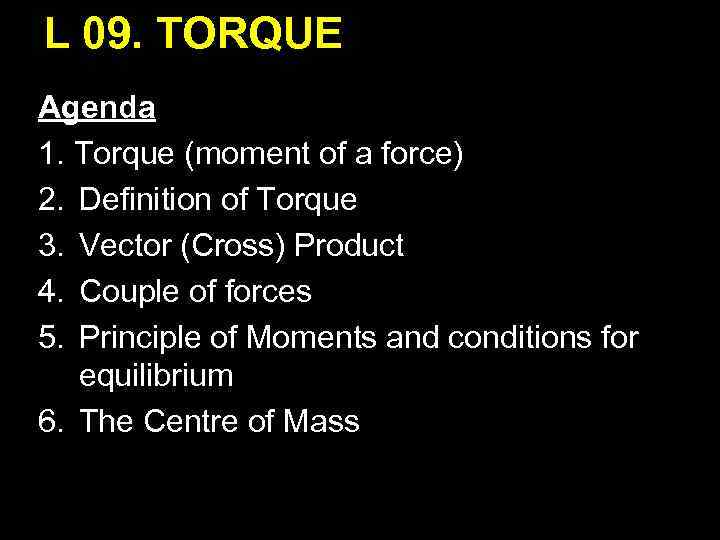L 09. TORQUE Agenda 1. Torque (moment of a force) 2. Definition of Torque 3. Vector (Cross) Product 4. Couple of forces 5. Principle of Moments and conditions for equilibrium 6. The Centre of Mass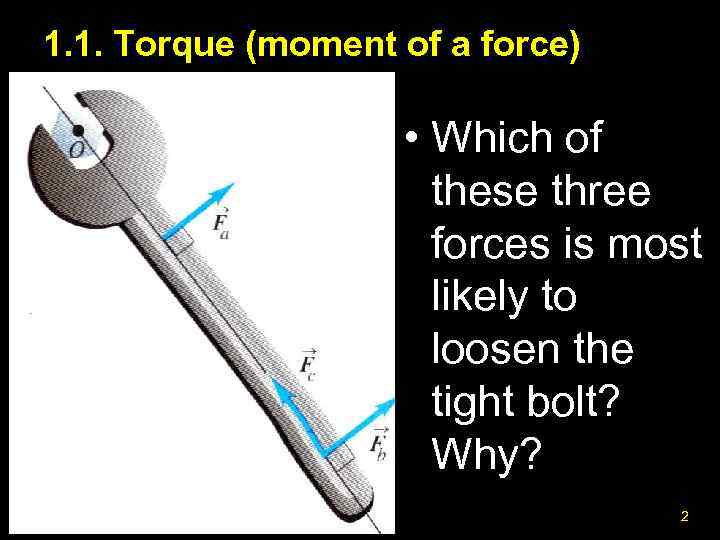1. 1. Torque (moment of a force) • Which of these three forces is most likely to loosen the tight bolt? Why? 2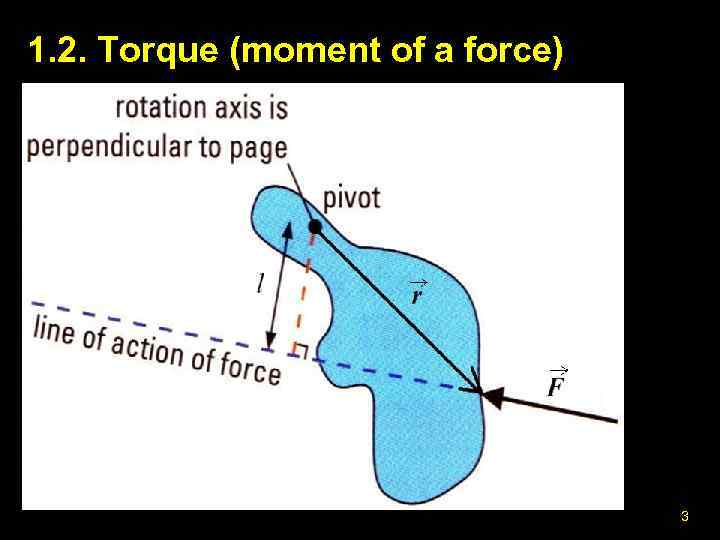1. 2. Torque (moment of a force) 3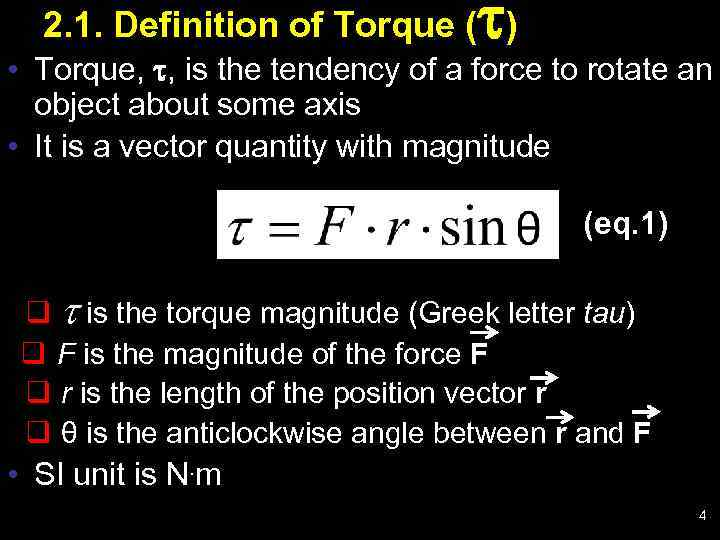2. 1. Definition of Torque ( ) • Torque, , is the tendency of a force to rotate an object about some axis • It is a vector quantity with magnitude (eq. 1) q t is the torque magnitude (Greek letter tau) q F is the magnitude of the force F q r is the length of the position vector r q θ is the anticlockwise angle between r and F • SI unit is N. m 4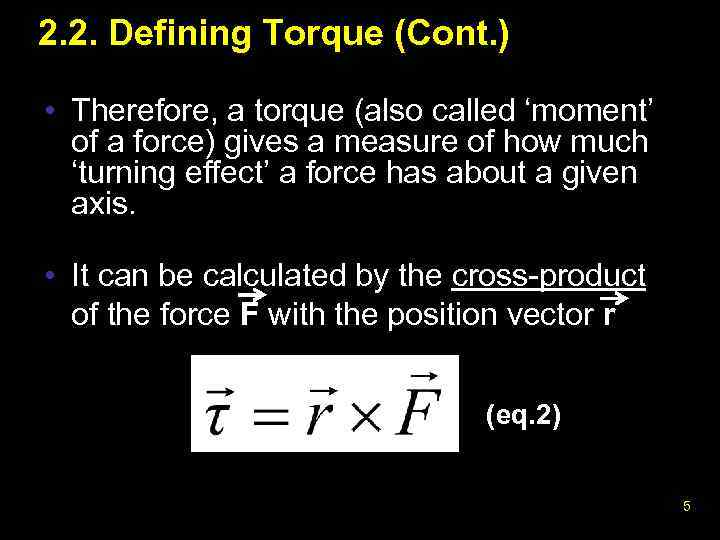2. 2. Defining Torque (Cont. ) • Therefore, a torque (also called ‘moment’ of a force) gives a measure of how much ‘turning effect’ a force has about a given axis. • It can be calculated by the cross-product of the force F with the position vector r (eq. 2) 5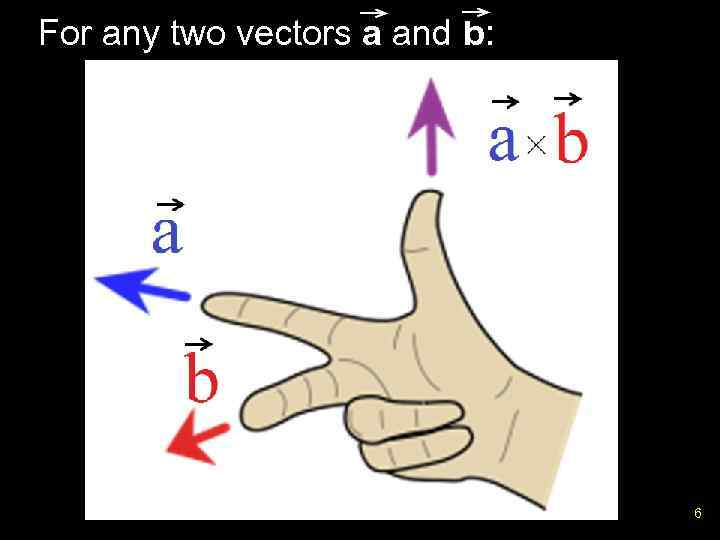For any two vectors a and b: 6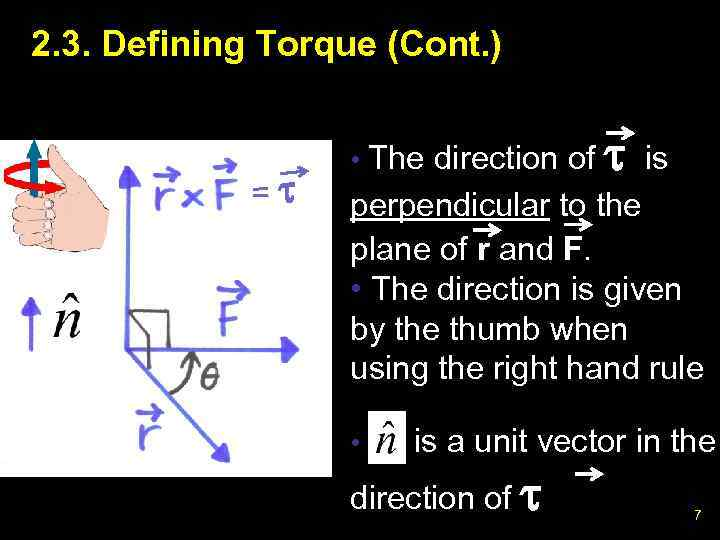2. 3. Defining Torque (Cont. ) = • The direction of is perpendicular to the plane of r and F. • The direction is given by the thumb when using the right hand rule • is a unit vector in the direction of 7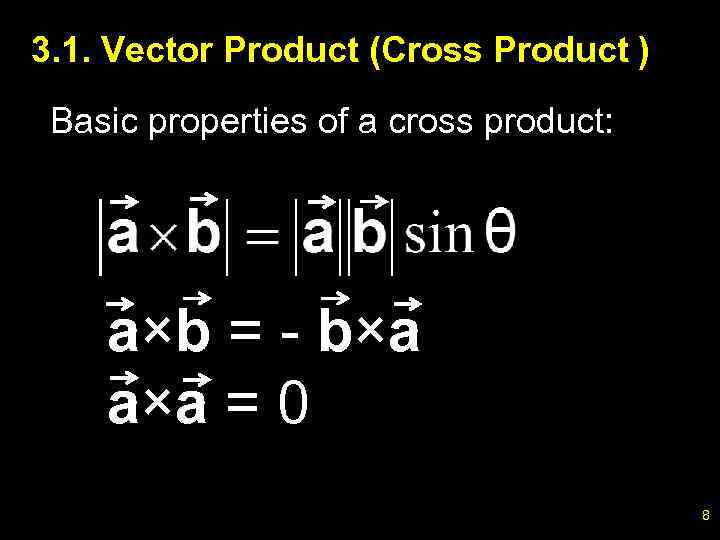3. 1. Vector Product (Cross Product ) Basic properties of a cross product: a×b = - b×a a×a = 0 8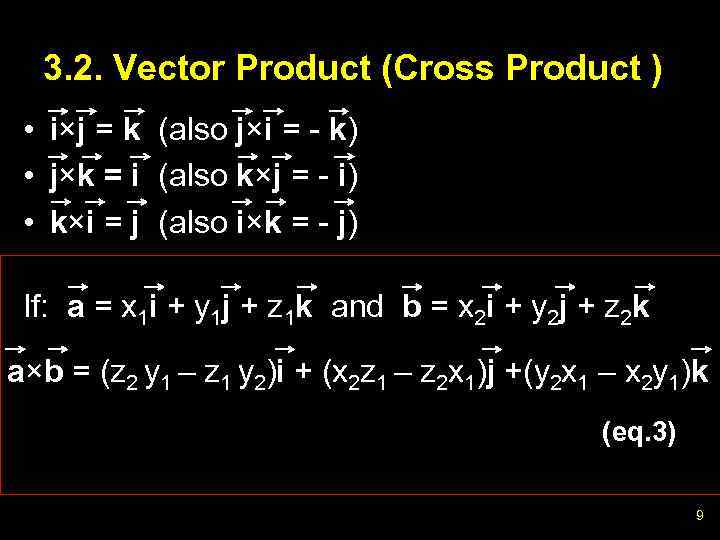3. 2. Vector Product (Cross Product ) • i×j = k (also j×i = - k) • j×k = i (also k×j = - i) • k×i = j (also i×k = - j) If: a = x 1 i + y 1 j + z 1 k and b = x 2 i + y 2 j + z 2 k a×b = (z 2 y 1 – z 1 y 2)i + (x 2 z 1 – z 2 x 1)j +(y 2 x 1 – x 2 y 1)k (eq. 3) 9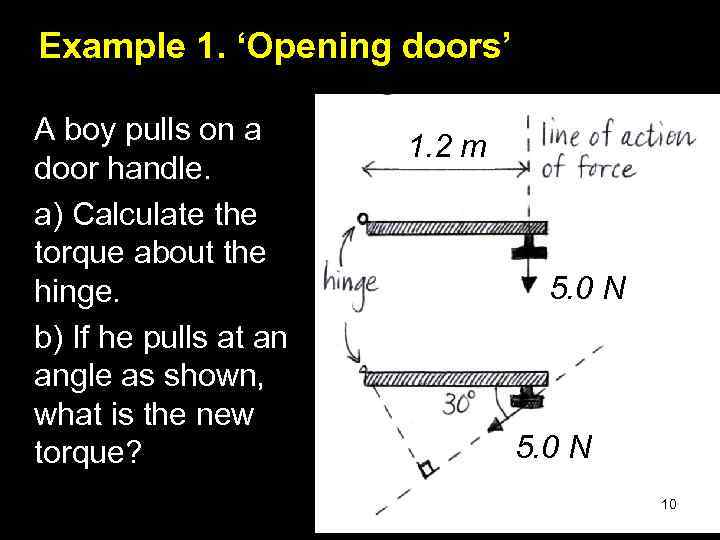Example 1. ‘Opening doors’ A boy pulls on a door handle. a) Calculate the torque about the hinge. b) If he pulls at an angle as shown, what is the new torque? 1. 2 m 5. 0 N 10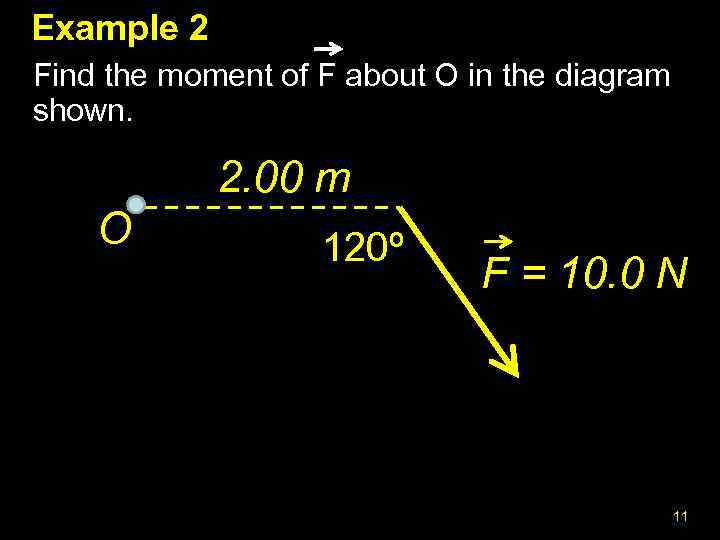Example 2 Find the moment of F about O in the diagram shown. 2. 00 m O 120º F = 10. 0 N 11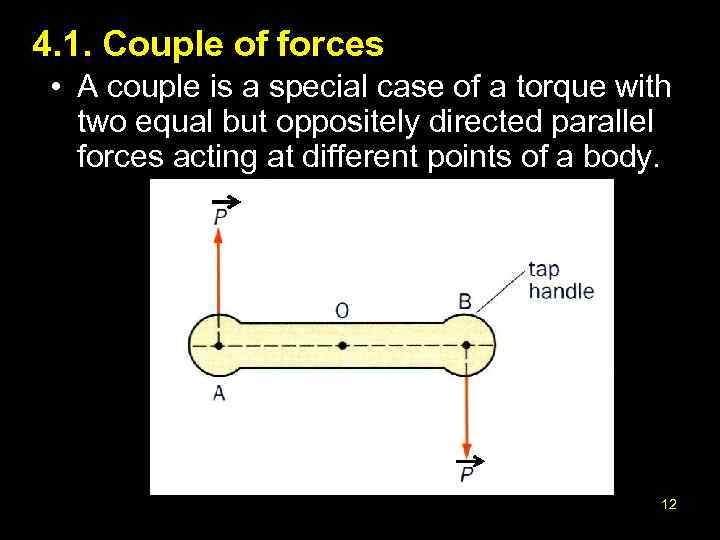4. 1. Couple of forces • A couple is a special case of a torque with two equal but oppositely directed parallel forces acting at different points of a body. 12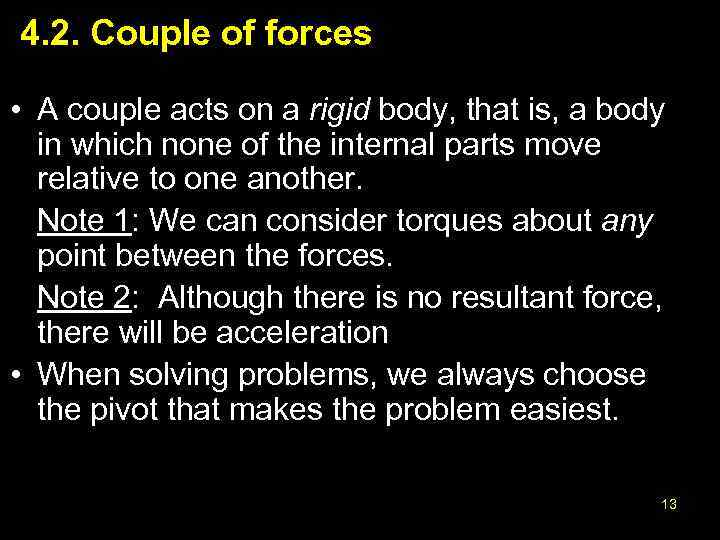4. 2. Couple of forces • A couple acts on a rigid body, that is, a body in which none of the internal parts move relative to one another. Note 1: We can consider torques about any point between the forces. Note 2: Although there is no resultant force, there will be acceleration • When solving problems, we always choose the pivot that makes the problem easiest. 13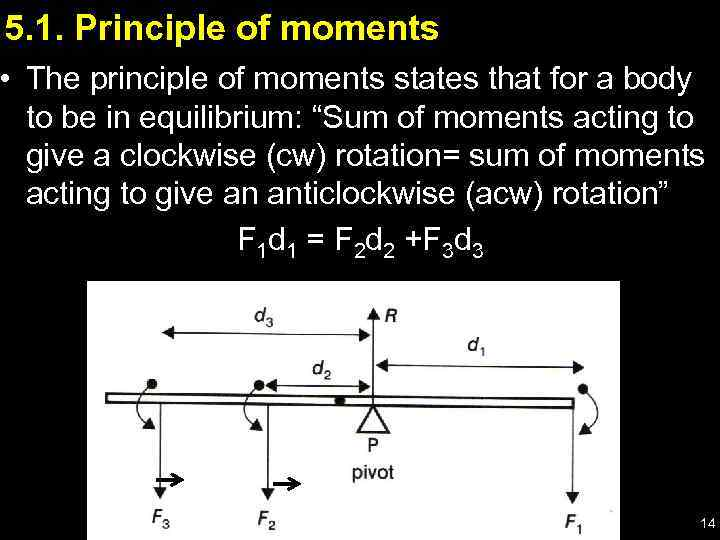5. 1. Principle of moments • The principle of moments states that for a body to be in equilibrium: “Sum of moments acting to give a clockwise (cw) rotation= sum of moments acting to give an anticlockwise (acw) rotation” F 1 d 1 = F 2 d 2 +F 3 d 3 14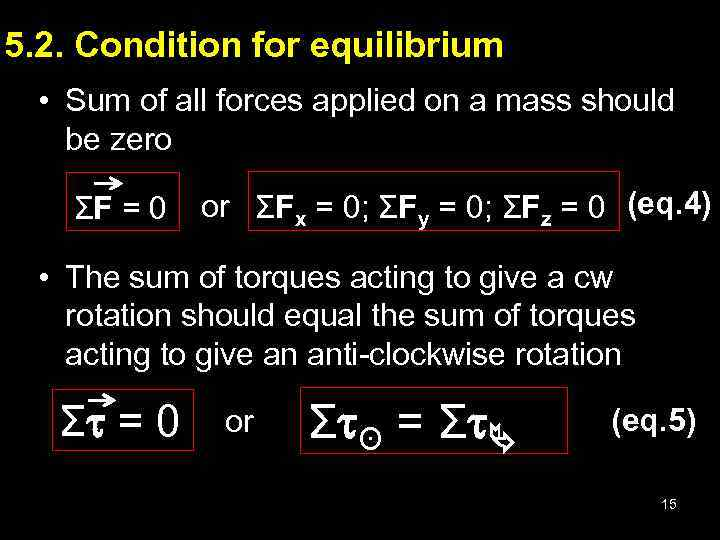5. 2. Condition for equilibrium • Sum of all forces applied on a mass should be zero ΣF = 0 or ΣFx = 0; ΣFy = 0; ΣFz = 0 (eq. 4) • The sum of torques acting to give a cw rotation should equal the sum of torques acting to give an anti-clockwise rotation Σ = 0 or Σ ʘ = Σ (eq. 5) 15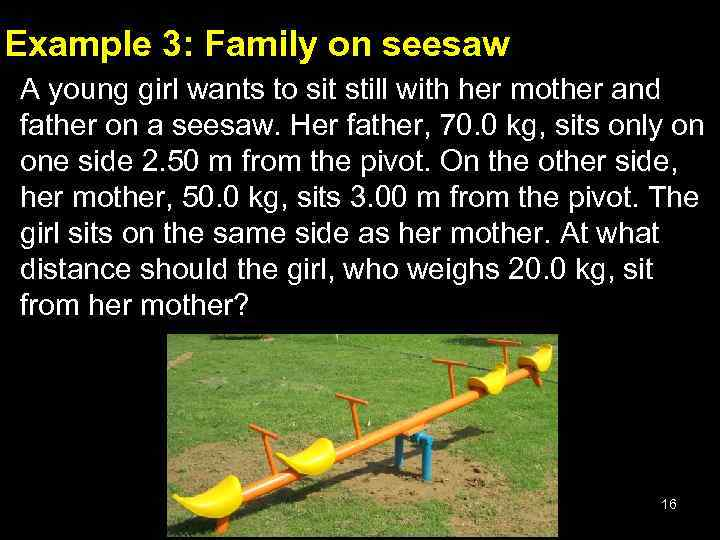Example 3: Family on seesaw A young girl wants to sit still with her mother and father on a seesaw. Her father, 70. 0 kg, sits only on one side 2. 50 m from the pivot. On the other side, her mother, 50. 0 kg, sits 3. 00 m from the pivot. The girl sits on the same side as her mother. At what distance should the girl, who weighs 20. 0 kg, sit from her mother? 16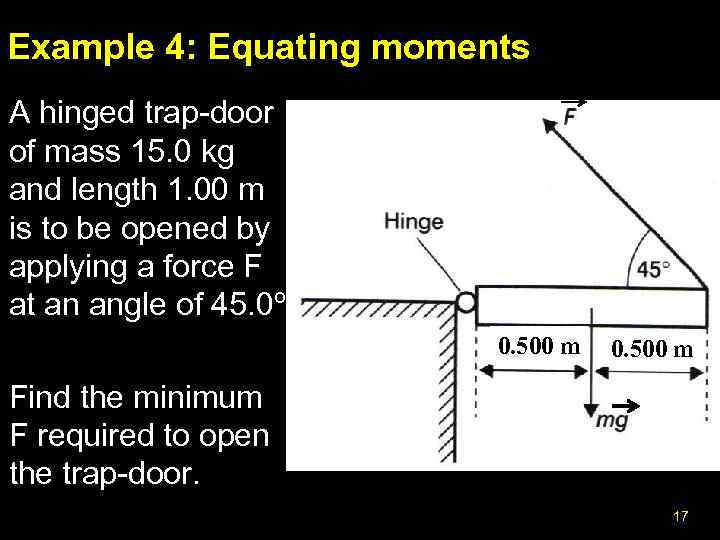Example 4: Equating moments A hinged trap-door of mass 15. 0 kg and length 1. 00 m is to be opened by applying a force F at an angle of 45. 0º. 0. 500 m Find the minimum F required to open the trap-door. 17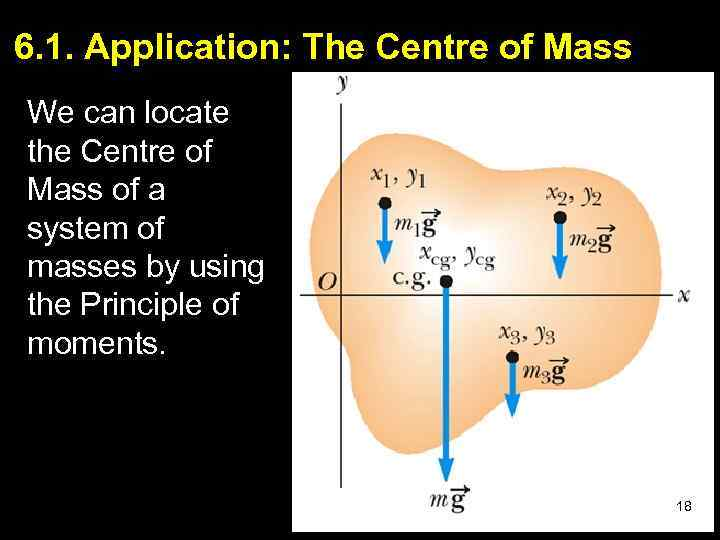6. 1. Application: The Centre of Mass We can locate the Centre of Mass of a system of masses by using the Principle of moments. 0. 500 m 1818 18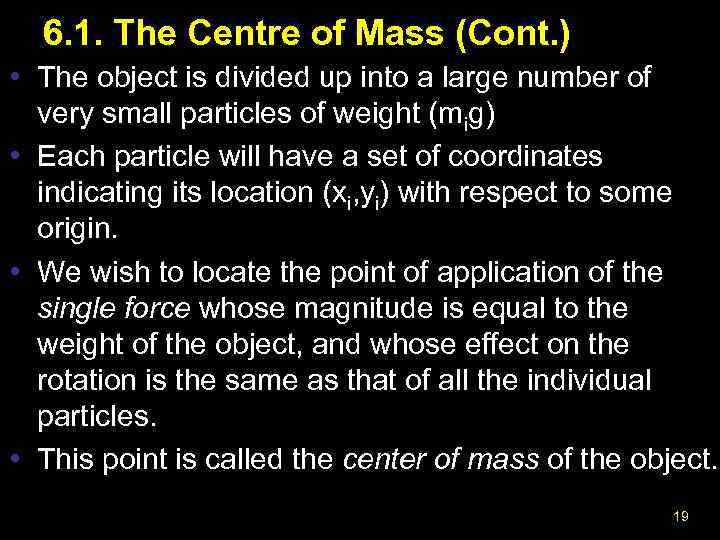6. 1. The Centre of Mass (Cont. ) • The object is divided up into a large number of very small particles of weight (mig) • Each particle will have a set of coordinates indicating its location (xi, yi) with respect to some origin. • We wish to locate the point of application of the single force whose magnitude is equal to the weight of the object, and whose effect on the rotation is the same as that of all the individual particles. • This point is called the center of mass of the object. 19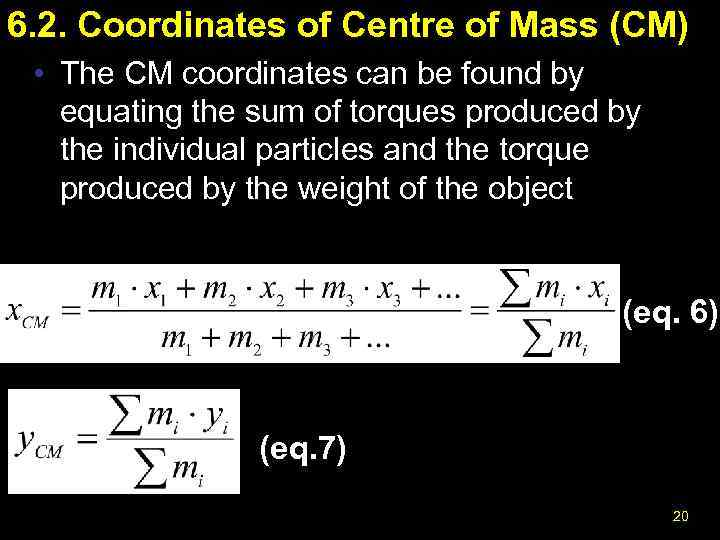6. 2. Coordinates of Centre of Mass (CM) • The CM coordinates can be found by equating the sum of torques produced by the individual particles and the torque produced by the weight of the object (eq. 6) (eq. 7) 20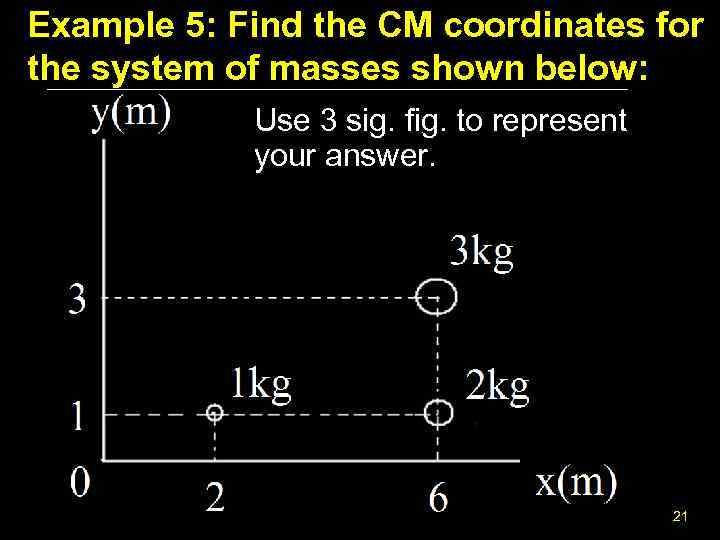Example 5: Find the CM coordinates for the system of masses shown below: Use 3 sig. fig. to represent your answer. 21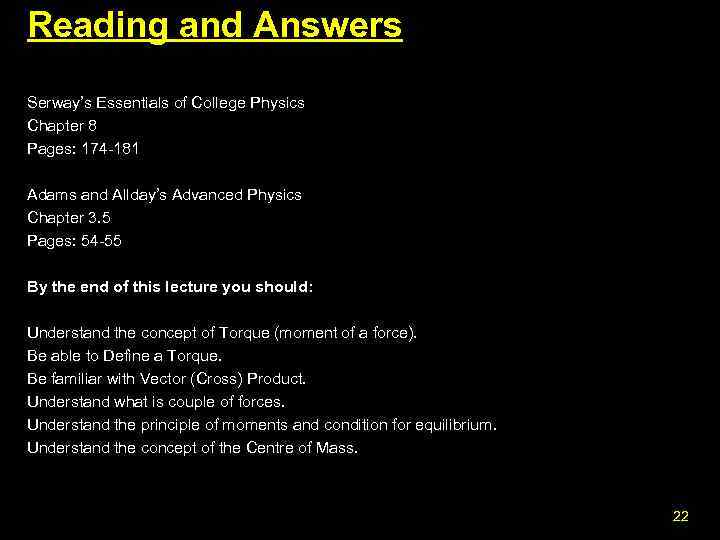Reading and Answers Serway’s Essentials of College Physics Chapter 8 Pages: 174 -181 Adams and Allday’s Advanced Physics Chapter 3. 5 Pages: 54 -55 By the end of this lecture you should: Understand the concept of Torque (moment of a force). Be able to Define a Torque. Be familiar with Vector (Cross) Product. Understand what is couple of forces. Understand the principle of moments and condition for equilibrium. Understand the concept of the Centre of Mass. 22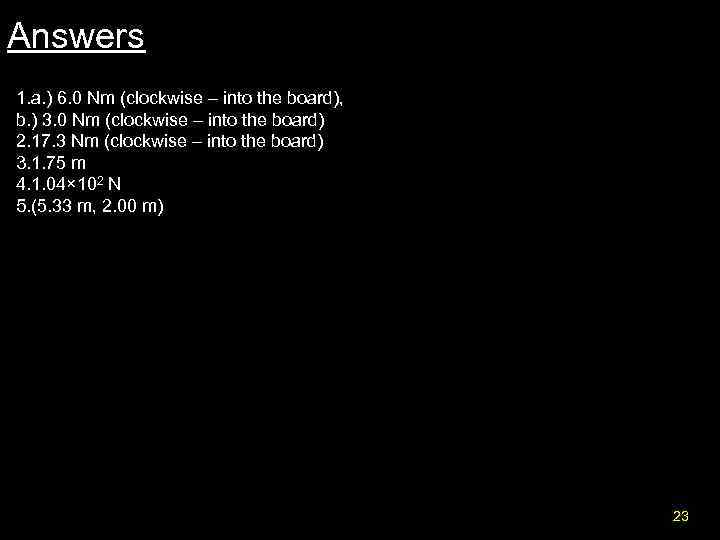Answers 1. a. ) 6. 0 Nm (clockwise – into the board), b. ) 3. 0 Nm (clockwise – into the board) 2. 17. 3 Nm (clockwise – into the board) 3. 1. 75 m 4. 1. 04× 102 N 5. (5. 33 m, 2. 00 m) 23